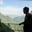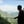Trusted answers to developer questions
Trusted Answers to Developer Questions

Related Tags

pandas
communitycreator

# What is pandas DataFrame.rpow() method?AKASH BAJWA

Grokking Modern System Design Interview for Engineers & Managers

Ace your System Design Interview and take your career to the next level. Learn to handle the design of applications like Netflix, Quora, Facebook, Uber, and many more in a 45-min interview. Learn the RESHADED framework for architecting web-scale applications by determining requirements, constraints, and assumptions before diving into a step-by-step design process.

### The pandas DataFrame.rpow() method

Here rpow() means reverse power. This is similar to the pow() method except that it calculates exponential power in a reversed order. If we want to use the power of x as the base and y as the exponent then the rpow() will calculate yx  instead of xy . This method provides exponential power to DataFrame values and others by applying an element-wise (binary operator floordiv) operation. This function is similar to other DataFrame functions, with the difference that it can replace missing data in one of the inputs.

### Syntax

DataFrame.rpow(other, axis = 'columns', level = None, fill_value = None)

### Parameters

It has the following argument values:

• other: This parameter is a single- or multiple-element data structure, or a list-like object. It can be a DataFrame, series, sequence, scalar, or a constant. It is a required parameter.
• axis: This is used for deciding the axis on which the operation is applied. It compares by index when set to 0 or ‘index’, and by columns when set to 1 or ‘columns’. This is an optional parameter.
• level: This parameter is used for broadcasting across a level and matching index values on the passed Multi-Index level. It can be an int or label. This is an optional parameter.
• fill_value: This parameter is a number or None. It specifies what to do with NaN values before subtracting. If data in both corresponding DataFrame locations is missing, the result will be missing. This is an optional parameter.

### Return value

This method returns the result of the arithmetic operation applied to the DataFrame.

### Applying a single scalar rpow() method on a DataFrame

Before applying the method, we require a DataFrame on which we can apply the method. To create a DataFrame, we first have to import pandas.

# importing pandas as pdimport pandas as pd# Creating a dataframe with two observationsdf= pd.DataFrame({"A":[10,5,1],				    "B":[6,2,3]})# Printing the dataframeprint(df)print()#reverse pow using scalar 5print(df.rpow(5))
Applying single scalar reverse power function

### Explanation

• Lines 4–5: We create a DataFrame df having dictionaries with key values A and B.
• Line 7: We print df.
• Line 10: We apply rpow() method on df  with a single scalar parameter 5.

Here the operation is performed in reverse as each value in df becomes the exponent of the scalar passed as a parameter.

### Applying the rpow() method on two DataFrames

Let us assume that we have two DataFrames df1 and df2. Each DataFrame is a set of dictionaries containing keys like A, B, and C. Each of the keys has 3 values.

# importing pandas as pdimport pandas as pd# Creating a dataframe with three observationsdf1 = pd.DataFrame({"A":[10,5,2],				    "B":[6,0,3],				    "C":[7,8,11]})# Print the dataframeprint(df1)print()# Creating another dataframe with three observationsdf2 = pd.DataFrame({"A":[2,3,5],				    "B":[6,3,2],				    "C":[12,10,0]})# Print the dataframeprint(df2)print()# applying rpow() on dataframesprint(df1.rpow(df2))
Applying the rpow() method on two dataframes

### Explanation

• Lines 4–6: We create a DataFrame df1 having dictionaries with key vales A, B, and C.
• Line 9: We print df1.
• Line 13–15: We create another DataFrame df2 having dictionaries with the same key values A, B, and C.
• Line 17: We print df2.
• Line 21: We apply the rpow() method on df1 passing df2 as an argument to it.

Since this operation operates in reverse, all the values of df1 become exponents of the values df2, element-wise. If we have some missing values in the data of either DataFrame, then we can simply use the parameter fill_value in addition to the other parameters by assigning it a value. This assigned value will be shown in all places wherever a value is missing.

RELATED TAGS

pandas
communitycreator

CONTRIBUTORAKASH BAJWA

Grokking Modern System Design Interview for Engineers & Managers

Ace your System Design Interview and take your career to the next level. Learn to handle the design of applications like Netflix, Quora, Facebook, Uber, and many more in a 45-min interview. Learn the RESHADED framework for architecting web-scale applications by determining requirements, constraints, and assumptions before diving into a step-by-step design process.

Keep Exploring

Learn in-demand tech skills in half the time